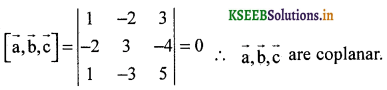# 2nd PUC Maths Question Bank Chapter 10 Vector Algebra

Students can Download 2nd PUC Maths Chapter 10 Vector Algebra Questions and Answers, Notes Pdf, 2nd PUC Maths Question Bank with Answers helps you to revise the complete Karnataka State Board Syllabus and to clear all their doubts, score well in final exams.

## Karnataka 2nd PUC Maths Question Bank Chapter 10 Vector Algebra

### 2nd PUC Maths Vector Algebra One Marks Questions and Answers

Question 1.
Compute the magnitude of the following vectors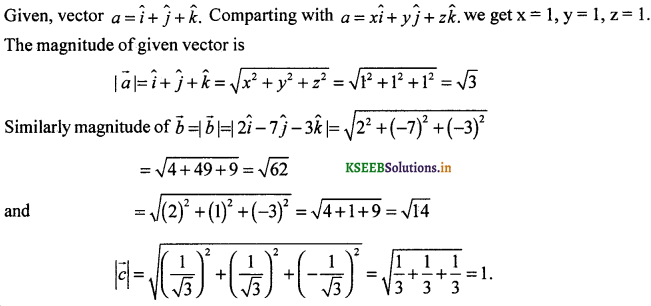Question 2.
Write two different vectors having same magnitude.
Two vectors can have same magnitude, if the sum of the squares of coefficient of î ĵ and k̂ is same. Let vectors a = (2î + 3ĵ + k̂) and b = (2î + 3ĵ + k̂) are different vectors having the same magnitude.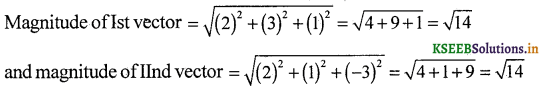i.e., they have same magnitude.

Question 3.
Write two different vectors having same direction.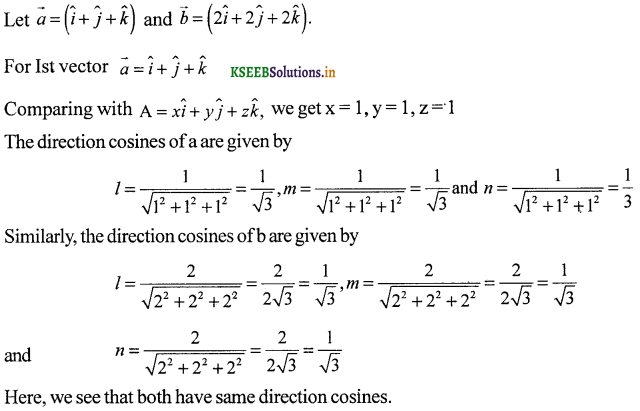Question 4.
Find the value of and x so that the vectors 2î + 3ĵ and xî + yĵ are equal.
Let a = 2î + 3ĵ and b = xî + yĵ be two given vectors.
Comparing coefficients of î and ĵ of both a and b, we have
⇒ x = 2 and y = 3.

Question 5.
Find the scalar and vector components of the vector with initial point (2,1) and terminal point (-5,7).
Vector with initial point A(2,1) and final (terminal) point B (-5,7) can be given by
AB = (x2 – x1)î + (y2 – y1)ĵ = (-5 – 2)î + (1 – 1)î = (-7)î + 6ĵ
Hence, the required scalar components (coefficients of î and ĵ) re -7 and 6 while the vector components are 7î and 6ĵ.### 2nd PUC Maths Vector Algebra Two Marks/Three Marks Questions and Answers

Question 1.
Find the sum of the vectors a = î – 2ĵ + k̂, b = -2î + 4ĵ + 5k̂ and c = î – 6ĵ – 7k̂
Here, given a = î – 2ĵ + k̂, b = -2î + 4ĵ + 5k̂, c = î – 6ĵ – 7k̂ Sum of these vectors can be calculated by adding their î, ĵ and k̂ components.Question 2.
Find the unit vector in the direction of the vector a = î + ĵ + 2k̂.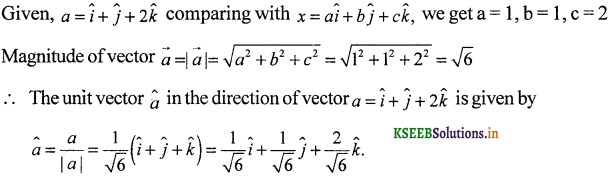Question 3.
Find the unit vector in the direction of vector $$\overrightarrow{\mathrm{PQ}}$$, where P and Q are the points (1, 2, 6). Q = (4, 5, 6) respectively.
The given points are P( 1,2,3) and Q (4,5,6).
∴ x1 = 1, y1 = 2, z1 = 3 and x2 = 4, y2 = 5, z2 = 6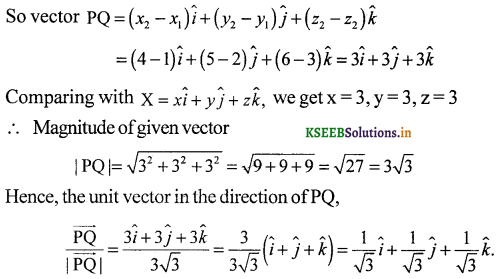Question 4.
For given vectors, a = 2î – ĵ + 2k̂ and b = -î + ĵ – k̂, find the unit vector in the direction of the vector a + b.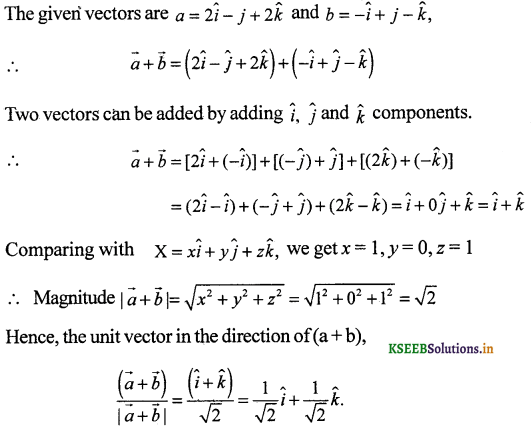Question 5.
Find a vector in the direction of vector 5î – ĵ + 2k̂ 2A which has magnitude 8 unit.
Let $$\vec{a}$$ = 5î – ĵ + 2k̂
Compring with X = xî – yĵ + zk̂, we get x = 5, y = -1 z = 2Question 6.
Show that the vectors 2î – 3ĵ + 4k̂ and -4î + 6ĵ – 8k̂ are collinear.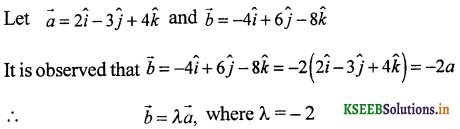Vectors a and b have the same direction, therefore they are collinear.Question 7.
Find the direction cosines of the vector î + 2ĵ + 3k̂.Question 8.
Find the direction cosines of the vector joining the points A (1,2,-3) and B (-1,-2, 1), directed from A to B.
The given points are A (1,2, -3) and B (-1,-2,1).
i.e., x1 = 1, y1 = 2, z1 =- 3 and x2 = – 1, z2 = 1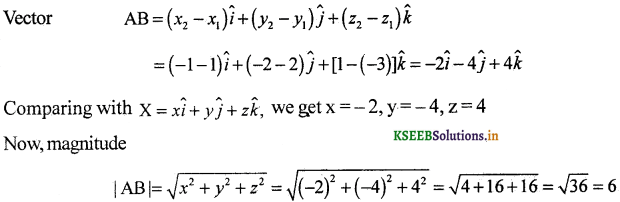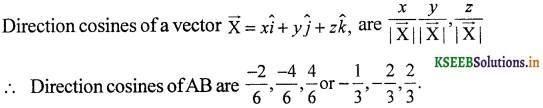Question 9.
Show tht the vector î + ĵ + k̂ is equally inclined to the axes OX, OY and OZ.
Let a = î + ĵ + k̂If a makes angles a, b and r respectively with (positive) OX, OY and OZ.
Then, we have cos α = 1 /√3
(∵ Direction cosines are the cosines of the angles made by î, ĵ, k̂ components of the vector with X, Y, and Z axes)Hence, the given vector a is equally inclined with OX, 0 Y and OZ.

Question 10.
Find the position vector of a point R which divides the line joining two points P and Q whose position vectors are î + 2ĵ – k̂ and -î + ĵ – k̂ respectively, in the ration 2 : 1
(i) internally
(ii) externally.
The position vector of a point R divided the line segment joining two points P and Q in the ratio m:n is given by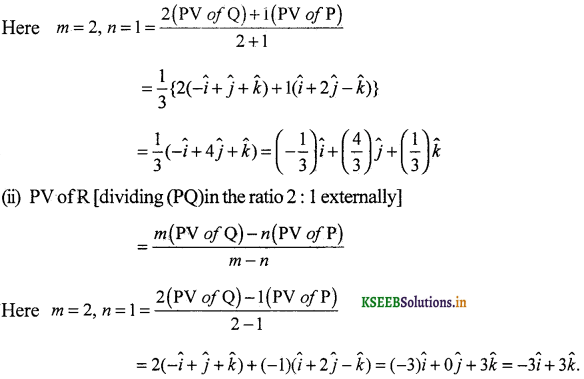Question 11.
Fnd the position vector of the mid-point of the vector joining points P (2,3,4) and Q(4,1,-2).
The position vector of mid-point of the vector joining the points P(2,3,4) and Q(4,1,-2) is given by,
PVof the mid-point of (PQ) = $$\frac { 1 }{ 2 }$$(PV of P + PVof Q)Question 12.
Show that the points A, B and C with position vectors, $$\vec{a}$$ = 3î – 4ĵ – 4k̂, $$\vec{b}$$ = 2î – ĵ + k̂ and $$\vec{c}$$ = î – 3ĵ – 5k̂ respectively, form the vertices of a right anled triangle.
Position vectors of points A, Band Care respectively given as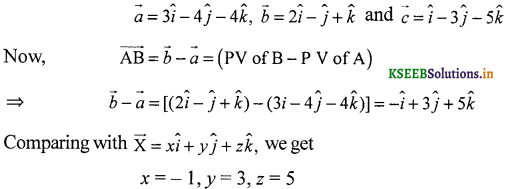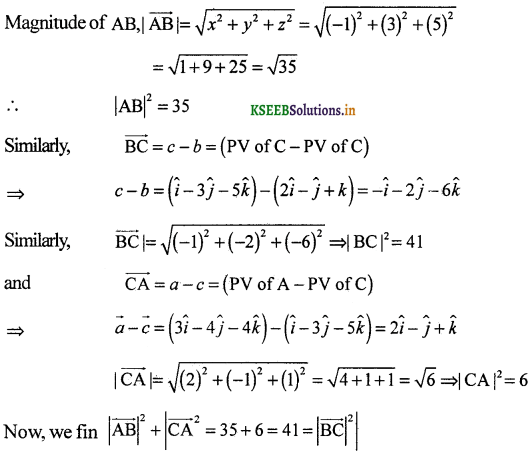Therefore, ∆ ABC is a right anged triangle with right angle at A.Question 13.
Find the angle between two vectors a and b with magnitudes √3 and 2 respectively, having a.b = √6.
It is given that |a| = √3, |b| = 2 and a.b = √6
Let θ be the required angle, thenHence, the angle between the given vectors a and b is π/4.

Question 14.
Find the angle between the vectors î – 2ĵ + 3k̂ and 3î – 3ĵ – 5k̂
Let $$\vec{a}$$ = î – 2ĵ + 3k̂ and $$\vec{b}$$ = 3î – 3ĵ – 5k̂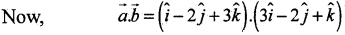= 1.3 + (-2).(-2) + 3.1 = 3 + 4 + 3 = 10
(Dot product of two vectors is equal to the sum of the products of their corresponding components.)
Let θ be the required angle between a and b, then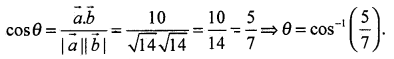Question 15.
Find the projection of the vector î – ĵ on the vector î + ĵ .
Let a = î – ĵ, b = î + ĵ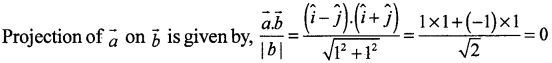Hence, the proection of vector $$\vec{a}$$ on $$\vec{b}$$ is 0.

Question 16.
Find the projection of the vector î + 3ĵ + 7k̂ A on the vector 7î – ĵ + 8k̂.Question 17.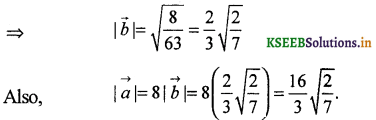Question 18.
Evaluate the product (3$$\vec{a}$$ – 5$$\vec{b}$$).(2$$\vec{a}$$ + 7$$\vec{b}$$).
We have, (3$$\vec{a}$$ – 5$$\vec{b}$$).(2$$\vec{a}$$ + 7$$\vec{b}$$)
= (3a).(2a + 7b) – (5$$\vec{b}$$).{2$$\vec{a}$$ + 7$$\vec{b}$$)
= 6 (a.a) + 21(a.b) – 10 (b.a) – 35 (b.b)
= 6 |a|2 + 11 (a.b) – 35 |b|2 (∵ a.a = |a|2 and a.b = b.a)

Question 19.
Find the magnitude of two vectors $$\vec{a}$$ and $$\vec{b}$$ having the same magnitude and such that the angle between them is 60° and their scalar product is 1/2.
Both vectors have same magnitude i.e.,|a| = |b|
and scalar product of vectors, $$\vec{a}$$.$$\vec{b}$$ = $$\frac { 1 }{ 2 }$$ (Given)
Let θ be the angle between two vectors a and b, then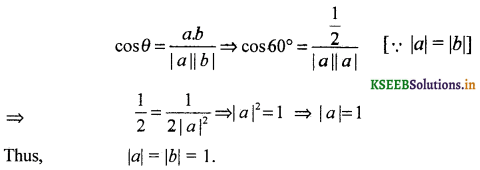Question 20.
Find |x|, if for a unit vector a, ($$\vec{x}$$ – $$\vec{a}$$).($$\vec{x}$$ + $$\vec{a}$$) = 12.
Given |a| = 1
(∵ It is a unit vector, magnitude of a unit vector is 1.)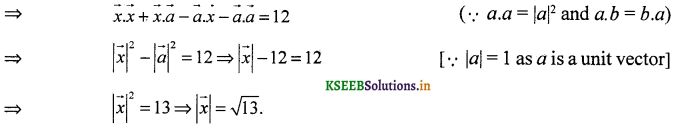Question 21.
If a = 2î + 2ĵ + 3k̂, b = -î + 2ĵ + k̂ and c = 3î + ĵ such that a + λb is perpendicular to c, then And the value of λ.
The given vectors are a = 2î + 2ĵ + 3k̂, b = -î + 2ĵ + k̂ and c = 3î + ĵ
Now, (a + λb) ⊥ c (Given)
⇒ (a + λb).c = 0
(∵ scalar product of two perpendicular vectors is zero)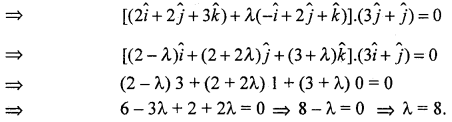Hence, the required value of λ is 8.

Question 22.
Show that |a| b + |b| a is perpendicular to |a| b – |b| a for any two non-zero vectors a and b.
Let p = |a| b + |b| a is perpendicular to |a|b – |b| a
Then p.q = (|a| b + |b|a).(|a| b – |b|a)
= |a|2 (b.b) – |a| |b| (b.a) + |b| |a| (a.b) – |b|2 (a.a)
= |a|2 |b|2 – |a| |b| (a.b) + |a| |b\ (a.b) – |b|2 |a|2 = 0
⇒ P ⊥ q (∵ If c.d = 0 ⇒ c is perpendicular to d)
Hence, |a| b + |b|a and |a|b – |b|a are perpendicular to each other for any two non-zero vectors a and b.

Question 23.
If a, b, c are unit vectors such that a + b + c = 0, then find the value of a.b + b.c + c.a.
Given, |a| = |b| – |c| = 1 and a + b + c = 0
We have (a + b + c).(a + b + c) = 0
⇒ a.(a + b + c) + b .(a + b + c) + c.(a + b + c) = 0
⇒ a.a + a.b + a.c + b.a + b.b + b.c + c.a + c.b + c.c = 0⇒ 3 + 2 (a.b + b.c + c.a) = 0
⇒ a.b + b.c + c.a = –$$\frac { -3 }{ 2 }$$.Question 24.
If either a = 0 or b = 0, then a.b = 0. But the converse need not to be true. Justify your answer with an example.
If a = 0 = 0î + 0ĵ + 0k̂ and b is non-zero i.e., b = xî + yĵ + zk̂
∴ a.b = (0î + 0ĵ + 0k̂) (xî + yĵ + zk̂) = (0 × t) + (0 × y) + (0 × z) = 0
So, if a = 0 or b = 0, then for same a.b = 0
To prove that converseneed not to be we have to prove that for two non-zero vectors a and’ b, a.b can be zero.Hence, the converse of the given statement need not be true.

Question 25.
If the vertices A, B, C of a triangle ABC have position vectors (1, 2, 3), (-1, 0, 0), (0, 1, 2) respectively then find ∠ABC (∠ABC si the angle between the vectors BA and BC).
We are given the points A(1, 2, 3), B(-1, 0, 0) and C(0, 1, 2).
Also, it is given that ∠ABC is the angle between the vectors BA and BC.
Here,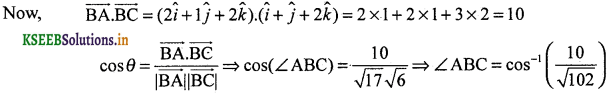Question 26.
Show that the points A(1, 2, 7), B(2, 6, 3) and C(3, 10, -1) are collinear.
The given points re A(1, 2, 7), B(2, 6,3) and C(3, 10, -1).∴ |AC| = |AB| + |BC|. Hence, the given points A, B and C are coliinear.Question 27.
Show that the vectors 2î – ĵ + k̂, î – 3ĵ – 5k̂ and 3î – 4ĵ – 4k̂ form the vertices of a right angled triangle.
Let 2î – ĵ + k̂, î – 3ĵ – 5k̂ and 3î – 4ĵ – 4k̂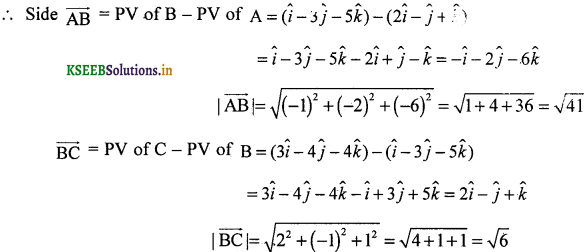Question 28.
Find $$|\vec{a} \times \vec{b}|$$, if a = î – 7ĵ + 7k̂ and b = 3î – 2ĵ + 2k̂
It is given that a = î – 7ĵ + 7k̂ and b = 3î – 2ĵ + 2k̂Question 29.
Find a unit vector perpendicular to each of the vectors $$\vec{a}+\vec{b}$$ and $$\vec{a}-\vec{b}$$, where $$\vec{a}$$ = 3î + 2ĵ + 2k̂ $$\vec{b}$$ = î + 2ĵ – 2k̂.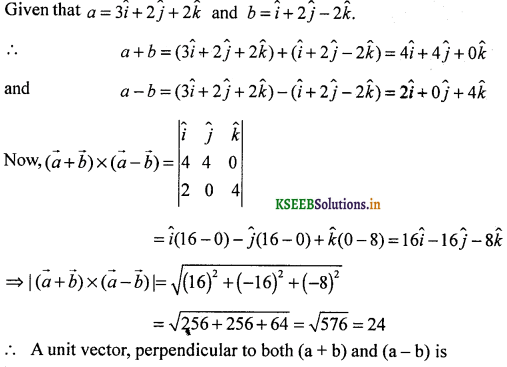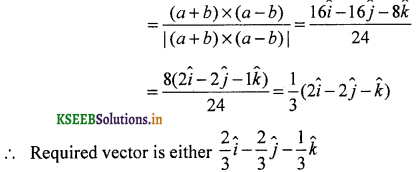Question 30.
If a unit vector $$\vec{a}$$, makes angle $$\frac{\pi}{3}$$ with î. $$\frac{\pi}{4}$$ with ĵ and an acute angle θ with k̂, then find θ and hence the components of a.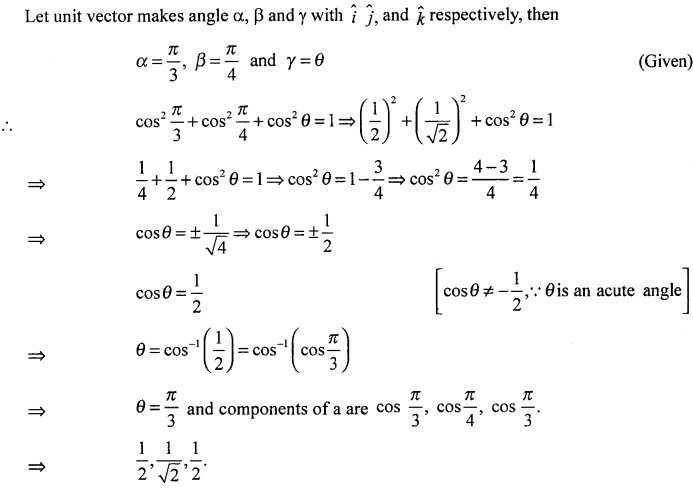Question 31.Question 32.
Find λ and u, if (2î + 6ĵ + 27k̂) × (î + λĵ + µk̂) = 0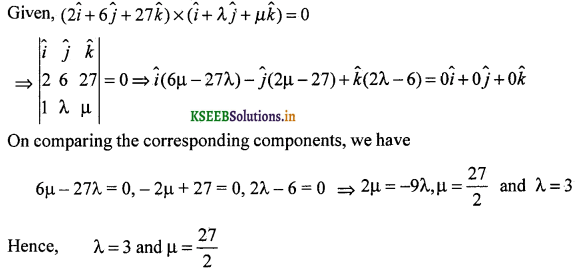Question 33.
Given that a.b = 0 and a × b = 0. What can you conclude about the vectors a and b?
Given that a.b = 0
Then, either |a| = 0 or |b| = 0 or a ⊥ b (in case a and b are non-zero) and if a × b = 0 then either |a| = 0 or |b| = 0or a||b (in case a and b are non-zero) But a and b cannot be perpendicular and parallel simulataneously.
Hence, |a| = 0 or |b| = 0.

Question 34.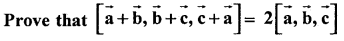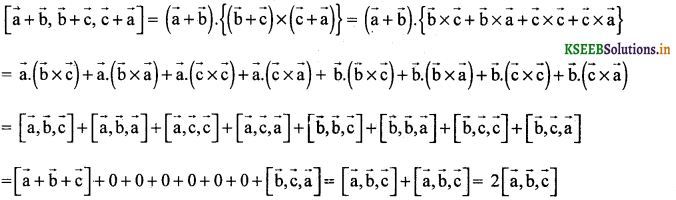Question 35.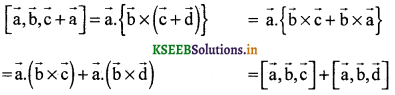Question 36.
Find the volume of the parallelopiped whose cotermius edges are 2î + ĵ + 3k̂, – î + 2ĵ + k̂ and 3î + ĵ + 2k̂.
Let $$\vec{a}$$ = 2î + ĵ + 3k̂, $$\vec{b}$$ = – î + 2ĵ + k̂ and $$\vec{c}$$ = 3î + ĵ + 2k̂.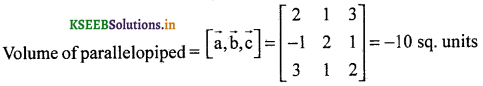$$\vec{a}$$ = î – 2ĵ + 3k̂, $$\vec{b}$$ = – 2î + 3ĵ – 4k̂ and $$\vec{c}$$ = î – 3ĵ + 5k̂are coplanar.